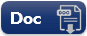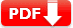# ASVAB Electronics Information Practice Test 2021 Question Answers

Free ASVAB Electronics Information Practice Test 2021 Question Answers PDF for Armed Services Vocational Aptitude Battery exam and Armed Forces Qualification Test (AFQT) exam preparation online.

Electronics Information (El) tests understanding Of electrical current, circuits, devices, and systems. Electronics information topics include electrical circuits, electrical and electronic systems, electrical currents, electrical tools, symbols, devices, and materials.

Actual Exam Content in EI Part

 Test Type Number of Question ASVAB Paper-and-Pencil 20-items CAT-ASVAB Test 16-items

## ASVAB Electronics Information Practice Test 2021

The following ASVAB Electronics Information Practice Test is only for reference purpose, it’s not an actual exam test questions. You can download our free ASVAB Electronics Practice Question in PDF (printable and editable), this will help you to make better ASVAB Test Prep offline. You can also use these files (PDF, DOC) as worksheets.

 Name of the Test ASVAB Practice Test ASVAB Stands for Armed Services Vocational Aptitude Battery AFQT Stands for Armed Forces Qualification Test Test Type Sample Multiple Choice Test Total Questions 20 (twenty) Answers and Explanation Available Printable and Editable PDF Available Subject Name Electronics Information (EI) Purpose Measures knowledge of electrical current, circuits, devices, and electronic systems

## ASVAB Electronics Practice Test 2021

Q1. One hertz is defined as

• A. one ampere per second.
• B. one cycle per second.
• C. one volt per second.
• D. one coulomb per second.

1 hertz is defined as 1 cycle per second. A 60-hertz electric current oscillates at 60 cycles per second.

Q2. One advantage of alternating current is that it is easy to

• A. store.
• B. convert into chemical energy.
• C. transport through wire.
• D. transmit through air.

Alternating current travels much further through wire than direct current.

Q3. Electric current can travel only through a

• A. wire.
• B. circuit.
• C. battery.

Without a circuit, electrons cannot travel, so there is no electric current.

Q4. Copper is used in most electric wires because it is

• A. a semiconductor.
• B. not likely to heat up during normal usage.
• C. a good resistor and inexpensive.
• D. a good conductor and inexpensive.

Copper is a good conductor and inexpensive. Choice B, while true, is not the whole answer, as it also applies, for example, to gold.

Q5. Amperes are a measure of

• A. the number of electrons moving through a conductor
• B. electrical pressure.
• C. a material’s ability to store electric current.
• D. the total resistance of a series circuit.

Amperes measure the number of electrons moving through a conductor.

Q6. Ohms are a measure of

• A. capacitance.
• B. resistance.
• C. current.
• D. cycles per second.
Answer: B Ohms are a measure of resistance.

Q7. If amperes = volts/ohms, then volts =

• A. 1/(amperes × ohms).
• B. ohms/amperes.
• C. amperes/ohms.
• D. amperes × ohms
Answer: D Using algebra, multiply both sides by ohms.
You should get volts = amperes × ohms.

Q8. A circuit with 20 amperes has a load of 12 ohms. What is the voltage?

• A. 60
• B. 120
• C. 240
• D. 24
Answer: C  volts = amperes × ohms.  = 20 × 12 =240.

Q9. If the resistance of a circuit is 0, the circuit is

• A. an open circuit.
• B. a short circuit.
• C. a superconductor circuit.
• D. a semiconductor circuit.

This circuit will have a dangerously high current and may burn up or cause a fire. It should trip a circuit breaker or blow a fuse before too
much damage occurs.

Q10. If a transformer raises the voltage, it will

• A. raise the resistance.
• B. reduce the resistance.
• C. raise the amperage.
• D. reduce the amperage.

The overall amount of power does not change. Power = amperes × volts. So if voltage rises, amperage must fall.

Q11. Which of these devices depends on the close relationship between electricity and magnetism?

• A. Transformer
• B. Electromagnet
• C. Electric motor
• D. All of the above

In a transformer, electricity is converted into magnetism and back into electricity. In an electromagnet, electricity is converted into magnetism. In an electric motor, electromagnets create a repulsive force that causes the rotor to spin.

Q12. If you are soldering two wires, you need solder,

• A. heat, and flux.
• B. and heat.
• C. and flux.
• D. flux, and electrical tape.

Heat alone will not do the job, because the wires will start to corrode when you heat them. The solder does not adhere to corrosion.

Q13. When connecting wires in the house, the bare copper wire is sometimes replaced by the

• A. the black wire.
• B. the white wire.
• C. the hot wire.
• D. conduit.

Conduit (either flexible or rigid) provides a safe return path for electricity in many wiring systems.

Q14. To hook up an electric heater, you need

• A. three supply wires.
• B. four supply wires.
• C. heavy supply wires.
• D. no supply wires.

Heaters use high current, which demands heavy wires.

Q15. In most home wiring, the hot wire is

• A. black.
• B. blue.
• C. green.
• D. white.

Black is the most common color for hot wires, although red and blue are sometimes used.

Q16. To control a light from each end of a hallway, you would install

• A. two single-pole switches.
• B. one four-way switch.
• C. one three-way switch.
• D. two three-way switches.

Three-way switches are used in pairs to control one light from two locations.

Q17. At 120 volts, a 15-ampere circuit will carry _______ power than a 20-ampere circuit.

• A. more
• B. less
• C. first less, then more
• D. first more, then less

Amperes = volts × power. Voltage does not change, so fewer amperes will carry less power.

Q18. To connect a battery properly, you must

• A. observe proper polarity.
• B. get the right voltage.
• C. both A and B.
• D. be certain the battery is chemical-free.

Both the polarity and the voltage must be correct for a battery to work right.

Q19. When you are making an electrical connection to a large battery,

• A. never tighten the terminal.
• B. tighten the terminal, then back off one turn.
• C. use salt water to prevent corrosion.
• D. tighten the terminal securely.

Loose electrical connections can cause fire or equipment damage. Choice C, saltwater, is wrong because it will cause corrosion, reducing
conductivity across the connection.

Q20. If you need to reduce the voltage in a certain part of a circuit, you could use a

• A. capacitor.
• B. transistor.
• C. resistor.
• D. inductor.
 Document Type Download Link Free Editable Doc FileFree Printable PDF File